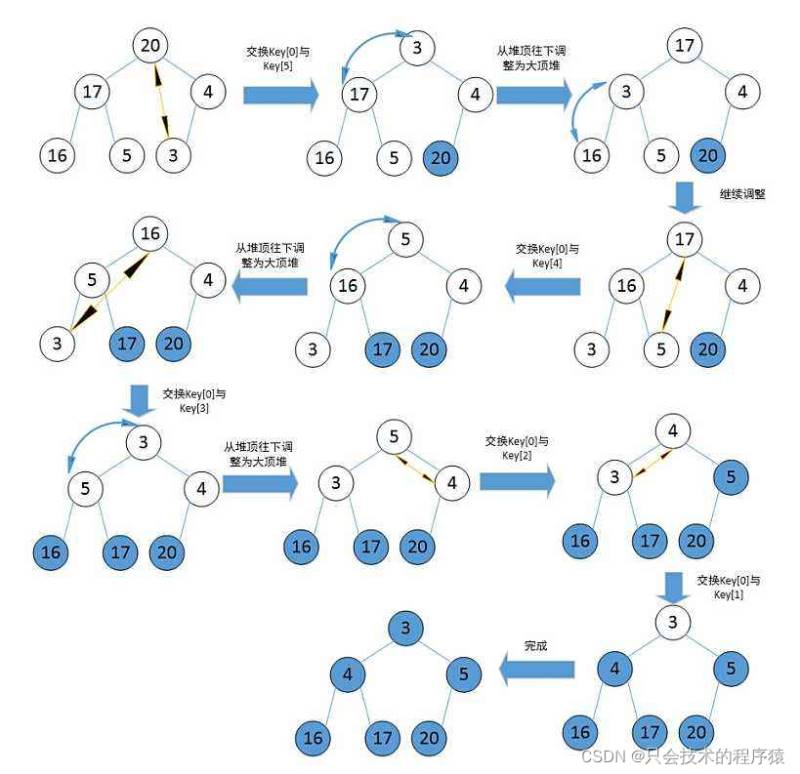﻿ java中几种常见的排序算法总结_java_脚本之家
java# java中几种常见的排序算法总结

## 本节目标；

：分析常见的比较排序算法基本原理及实现

：分析排序算法的性能分析

：分析Java中常用排序方法

1 排序

排序，就是使一串记录，按照其中某个或某些关键字的大小，递增或递减排列的操作。

2 稳定性

两个相同的数据，如果经过排序后，排序算法能保证其相对位置不发生变化，则称该算法具备稳定发行。## 【插入排序】## 【优化版】

分析步骤；

``` public static void insertSort(int[] array) {
for (int i = 1; i < array.length; i++) {
int tmp = array[i];
int j = i-1;
for (; j >= 0 ; j--) {
if(array[j] > tmp) {
array[j+1] = array[j];
}else {
//array[j+1] = tmp;  只要j回退的时候，遇到了 比tmp小的元素就结束这次的比较
break;
}
}
//j回退到了 小于0 的地方
array[j+1] = tmp;
}
}```

## 【希尔排序】

(以了解为主 面试很少考的）【分析步骤 】## 【选择排序】

分析步骤；## 【堆排序】``` public static void createHeap(int[] array) {
for (int parent = (array.length - 1 - 1) / 2; parent > 0; parent--) {//设置出父亲节点
shiftDown(array, parent, array.length);//
}
}
public static void shiftDown(int[] array, int parent, int len) {
int child = 2 * parent + 1;//设置出子节点
while (child < len) {
if (child + 1 < len && array[child] < array[child + 1]) {
child++;//以防有两个子节点 找出两个子节点最大一位
}
if (array[child] > array[parent]) {
swap(array, parent, child);//交换父亲节点和子节点
parent = child;//从新设置父亲节点
child = 2*parent+1;
}else{
break;
}
}
}
public static void heapSort(int[] array) {//
createHeap(  array);
int end  = array.length-1;//每次交换完最后一位元素减一位
while(end > 0){
swap(array,0,end);
shiftDown(array,0, array.length-1);//这个循环里 向下转型
end--;
}
}```

## 【冒泡排序】## 介绍一个冒泡排序的优化方法；## 【快速排序】

1   从待排序区间选择一个数，作为基准值（pivot）

2   partition：遍历整个待排序区间， 将比基准小的(可以与基准相同)放在基左侧，将比基准大                   的(可以与基准相同）放在基准右侧。

3   采用分治思想，对左右两个小区间按照同样的方法进行处理  直到小区间的长度 == 1； 则其                 代表有序，如果长度 ==0， 则代表没有元素。``` public static void quick(int[] array,int left,int right) {
if( left >= right) {//判断递归条件 不满足退出
return;
}
int pivot =  partition( array,left,right);//找出基准值
quick( array, left, pivot-1);//左侧进行递归
quick( array, pivot+1 ,right);//右侧进行递归
}
private static int partition(int[] array,int start,int end) {
int tmp = array[start];
while(start< end){//
while(start< end &&array[end] >  tmp){//末尾值比tmp大时 将末尾下标减减
end--;
}
array[start] = array[end];//在右侧遇到比tmp小的元素 进行交换
while(start< end && array[start] < tmp ){//先从头部进行比较 比tmp小时交换
start++;
}
array[end] = array[start];
}
array[end] = tmp;
return end;
}
public static void quickSort(int[] array) {
quick(array,0, array.length-1);
}
}
}```

## 【归并排序】

归并排序（MERGE-SORT）是建立在归并操作上的一种有效的排序算法，该算法采用分治法（Divide and Conquer)的一个非常典型的应用。将以有序的子序列合并，得到完全有序的序列；即先使每个子序列有序，再使子序列段间有序。若将两个有序表合并成一个有序表，称为二路归并。

```public static int[] mergeArray(int[] array1,int[] array2) {
//注意判断参数
int [] tmp = new int[array1.length + array2.length];
int i = 0;
int s1 = 0;
int e1 = array1.length-1;
int s2 = 0;
int e2 = array2.length-1;
while( s1<e1 && s2<e2){
if(array1[s1] < array2[s2]){
tmp[i++] = array1[s1++];

}
if(array1[s1] > array2[s2]){
tmp[i++] = array2[s2++];
}
}
while( s1<= e1){
tmp[i++] = array1[s1++];
}
while( s2<= e2){
tmp[i++] = array2[s2++];
}
return tmp;```

## 【正文】## 【代码简介；】

``` public static void mergeSort1(int[] array) {

mergeSortInternal(array,0,array.length-1) ;

}
private static void mergeSortInternal(int[] array,int low,int high) {
if(low > high){
return ;
}
int mid  = (low +(high-low))/2;//找到数组的中间位置 分为左右两部分
mergeSortInternal( array, low,mid-1 );// 将左边部分进行递归排序
mergeSortInternal( array, mid+1,high );// 将右边部分进行递归排序
merge(array,low,mid,high);// 将两部分进行合并

}
private static void merge(int[] array,int low,int mid,int high) {
int [] tmp = new int[array.length];//创建一个新的数组
int s1 = low;
int e1 = mid;
int s2 = mid+1;
int e2 = high;
int i = 0;
while(s1<e1 && s2<e2){//循环的的条件
if(array[s1] < array[s2]){
tmp[i++] =  array[s1++];
}else{
tmp[i++] = array[s2++];
}

}
while(s1 < s2){//当到这一步 说明左侧比右侧长   将左侧剩余部分衔接到数组中
tmp[i++] = array[s1++];
}
while(s2 < e2){
tmp[i++] =  array[s2++];//当到这一步 说明左侧比右侧长   将左侧剩余部分衔接到数组中

}
for(int j = 0; j < i; j++){//将tmp数组中元素放置array数组中
array[i+low] = tmp[j];

}

```

## 【排序总结】